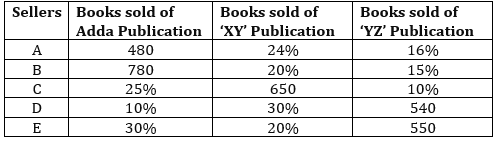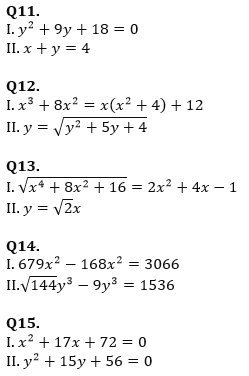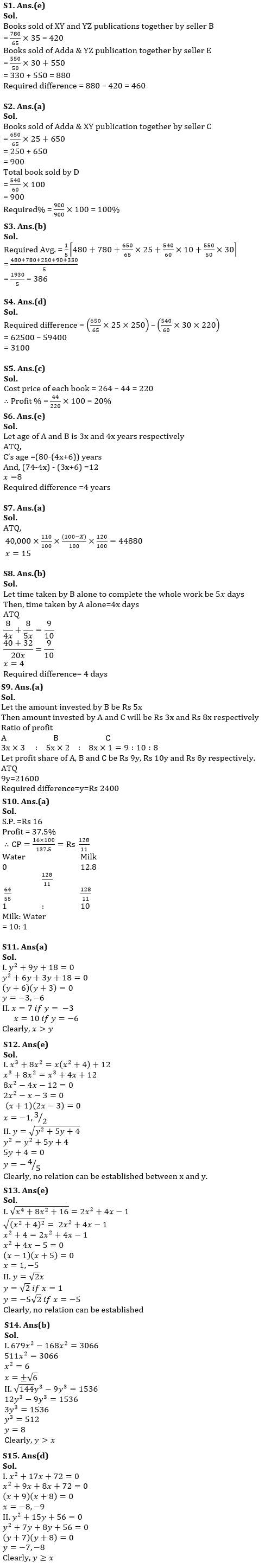Latest Banking jobs   »

# Quantitative Aptitude Quiz For IBPS RRB PO/Clerk Mains 2022- 10th September

Directions (1-5): Study the table carefully and answer the questions.
Table given below shows percentage of books sold of 3 different publications by five different sellers in a month.
Note: Books are sold by three publications only.Q1. Books sold by seller B of XY and YZ publications together is how much more/less than books sold by E of Adda & YZ publications together?
(a) 360
(b) None of these
(c) 380
(d) 420
(e) 460

Q2. Books sold by seller C of Adda & XY together is what percent of total books sold by seller D ?
(a) 100%
(b) 80%
(c) None of these
(d) 150%
(e) 120%

Q3. What is average number of books sold by all sellers of Adda publication?
(a) 392
(b) 386
(c) 406
(d) None of these
(e) 414

Q4. If selling price of each book of Adda publication sold by seller C is Rs. 250 and selling price of each book of XY publication sold by seller D is Rs. 220. Then find the difference in selling price of books of Adda publication sold by C and XY publication sold by D?
(a) Rs. 4500
(b) Rs. 2900
(c) Rs. 3600
(d) Rs. 3100
(e) Rs. 4200

Q5. If profit made on each book sold by seller E is Rs. 44. Then find profit percent of each book sold by seller E? (Given that selling price of each book is Rs. 264)
(a) 22%
(b) 25%
(c) 20%
(d) 15%
(e) 30%

Q6. Ratio of age of A and B, 6 years ago was 3: 4. Sum of the present age of B and C is 80 years. C is 12 years elder to A. Find the difference of B and C’s age five years later?
(a) 3 years
(b) 7 years
(c) 6 years
(d) 5 years
(e) 4 years

Q7. A man invested Rs 40,000 in mutual fund for 3 years. In first year, his amount is increased by 10%, in 2nd year his amount is decreased by X% and in 3rd year, his amount is again increased by 20% and he got Rs 4880 more at the end. Find value of X?
(a) 15
(b) 12.5
(c) 20
(d) 13 ⅓
(e) 17 ½

Q8. A alone takes 20% less time than that of B alone to do a work. When they together work for 8 days, 90% of the work gets completed. Find difference between their individual time to do the whole work?
(a) 4 ½ days
(b) 4 days
(c) 5 days
(d) 6 days
(e) 8 days

Q9. Investment made by A and C is 40% less and 60% more than that of B respectively and ratio of period of investment of A, B and C are 3:2:1. If average of their profit is Rs 21600 then find difference between A’s and C’s profit share (in Rs.).
(a) 2400
(b) 2000
(c) 1800
(d) 2600
(e) 2800

Q10. A milkman pays Rs. 12.8 per liter of milk. He added water to it and sells the mixture at Rs. 16 per liter at a profit of 37.5%. Find the ratio of milk to that of water added to the mixture?
(a) 10: 1
(b) 1: 10
(c) 11: 1
(d) 5: 1
(e) 10: 3

Directions (11-15): In each of these questions, two equations I and II are given. You have to solve both the equations and give answer.
(a) x > y
(b) x < y
(c) x ≥ y
(d) x ≤ y
(e) x = y or no relation can be established between x and ySolutions#### Congratulations!Download Hindu Review of October 2021: Free PDF